+
Solving single-step equations
Solving Linear Equations and Inequalities
0
of 0 possible points

# Solving single-step equations

Author: Sophia Tutorial
##### Description:

Identify the operation needed to solve a single-step equation.

(more)

Sophia’s self-paced online courses are a great way to save time and money as you earn credits eligible for transfer to many different colleges and universities.*

No credit card required

46 Sophia partners guarantee credit transfer.

299 Institutions have accepted or given pre-approval for credit transfer.

* The American Council on Education's College Credit Recommendation Service (ACE Credit®) has evaluated and recommended college credit for 33 of Sophia’s online courses. Many different colleges and universities consider ACE CREDIT recommendations in determining the applicability to their course and degree programs.

Tutorial
what's covered
1. Solving single-step equations
2. Subtraction Problems
3. Multiplication Problems
4. Division Problems

# 1. Solving single-step equations

Solving linear equations is an important and fundamental skill in algebra. In algebra, we are often presented with a problem where the answer is known, but part of the problem is missing. The missing part of the problem is what we seek to find. An example of such a problem is shown below.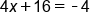Notice the above problem has a missing part, or unknown, that is marked by x. If we are given that the solution to this equation is −5, it could be plugged into the equation, replacing the x with −5. This is shown in below: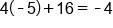Multiply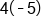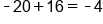Add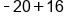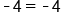True!

Now the equation comes out to a true statement! Notice also that if another number, for example, 3, was plugged in, we would not get a true statement.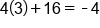Multiply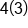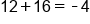Add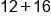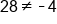False!

Due to the fact that this is not a true statement, this demonstrates that 3 is not the solution. However, depending on the complexity of the problem, this “guess and check” method is not very efficient. Thus, we take a more algebraic approach to solving equations. Here we will focus on what are called “one-step equations” or equations that only require one step to solve. While these equations often seem very fundamental, it is important to master the pattern for solving these problems so we can solve more complex problems.

To solve equations, the general rule is to do the opposite. For example, consider the following example.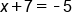The 7 is added to the x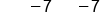Subtract 7 from both sides to get rid of it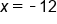Our Solution

Then we get our solution, x = − 12. The same process is used in each of the following examples.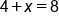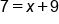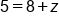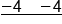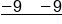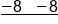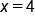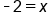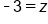1b. Subtraction Problems

In a subtraction problem, we get rid of negative numbers by adding them to both sides of the equation. For example, consider the following example.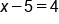The 5 is negative, or subtracted from z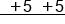Add 5 to both sides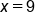Our Solution

Then we get our solution x = 9. The same process is used in each of the following examples. Notice that each time we are getting rid of a negative number by adding.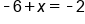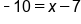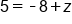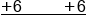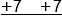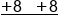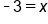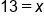1c. Multiplication Problems

With a multiplication problem, we get rid of the number by dividing on both sides. For example consider the following example.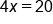Variable is multiplied by 4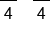Divide both sides by 4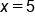Our Solution

Then we get our solution x=5

With multiplication problems it is very important that care is taken with signs. If x is multiplied by a negative then we will divide by a negative. This is shown in the next example: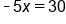Variable is multiplied by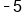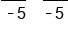Divide both sides by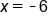Our Solution

The same process is used in each of the following examples. Notice how negative and positive numbers are handled as each problem is solved.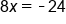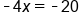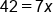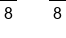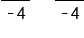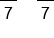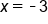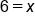1d. Division Problems

In division problems, we get rid of the denominator by multiplying on both sides. For example consider our next example.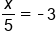Variable is divided by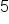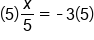Multiply both sides by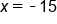Our Solution

Then we get our solution x = − 15. The same process is used in each of the following examples.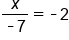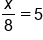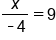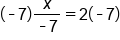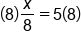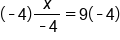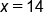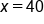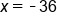The process described above is fundamental to solving equations. Once this process is mastered, the problems we will see have several more steps. These problems may seem more complex, but the process and patterns used will remain the same.

big idea
To solve single-step equations, first identify the operation being applied to the variable. To isolate the variable, apply the inverse operation. Addition and subtraction are inverses of each other; multiplication and division are inverses of each other.

summary
Solving single-step equations involves isolating the variable that you're trying to solve for by using an inverse operation. Any operation that you do on one side of the equation needs to be done on the other side. You can always check your solution by substituting it in for the variable in your original equation and seeing if the statement holds true. This is good practice to get into when you're doing simple equations because when you do things more complicated, it's more likely that you're going to be making a mistake.

Source: Adapted from "Beginning and Intermediate Algebra" by Tyler Wallace, an open source textbook available at: http://wallace.ccfaculty.org/book/book.html

Terms to Know
Equation

a mathematical statement that two quantities or expressions are equal in value

Rule of Equality

any operation performed on one side of the equation must be performed on the other side, in order to keep quantities equal in value

Rating